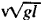# Wave Drag

Also found in: Wikipedia.
The following article is from The Great Soviet Encyclopedia (1979). It might be outdated or ideologically biased.

## Wave Drag

(in acoustics), for a gaseous or liquid medium, the ratio of the sound pressure p in a plane traveling wave to the vibration velocity v of the particles in the medium. The wave drag characterizes the degree of stiffness of the medium—that is, the medium’s ability to resist the formation of distortion—in the traveling-wave mode. It is independent of the wave shape and is expressed by the formula pic = pc, where p is the density of the medium and c is the speed of sound. Wave drag is the acoustic impedance of the medium for plane waves. The term“wave drag” was introduced by analogy with the terminology used in the theory of electrical transmission lines; here pressure corresponds to voltage, and the displacement velocity of the particles corresponds to electrical current.

Wave drag is a very important characteristic of mediums; it determines reflection and refraction conditions for waves at the interface. When a plane wave is normally incident on a plane interface between two mediums, the reflection coefficient is a function only of the ratio between the wave drags of these mediums. If they are equal, the wave passes through the interface without reflection. The concept of wave drag may also be applied to solids (for longitudinal and trans-verse elastic waves in an infinite solid body and for longitudinal waves in a rod) by defining it as the ratio of the corresponding mechanical stress, taken with a reversed sign, to the particle velocity of the medium.

K. A. NAUGOL’NYKH

## Wave Drag

in hydroaeromechanics.

(1) In gas dynamics, wave drag is the additional aerodynamic drag that results when the velocity of a gas relative to a body exceeds the speed of propagation in the gas of weak (acoustic) disturbances—that is, in cases of supersonic flow. Wave drag, which is the result of expenditures of energy for the formation of shock waves, is several times greater than the drag caused by friction and the formation of vortices. The wave drag coefficient increases sharply as the speed of the body v approaches the speed of sound c in the medium—in other words, as the Mach number M — vie approaches 1. The strength of wave drag depends on the shape of the body, the angle of attack, and the Mach number.

(2) Wave drag in a heavy liquid is one of the component forces of the resistance of a fluid to the movement of bodies. It arises during the movement of a body near the free surface of a heavy liquid or the interface of liquids with different densities. It is caused by the formation on the surface of the liquid of waves created by the moving body, which is performing work in overcoming the reaction of the liquid; this reaction is the force of wave drag. The value of the wave drag depends on the shape of the body, the depth of its submersion under the free surface, and the speed of its movement, as well as on the depth and width of the channel in which the movement occurs. The formation of waves upon motion of the body depends on the Froude number, Fr =(where v is the speed of movement of the body, l is its length, and g is the acceleration of gravity), which is a similarity criterion for the simulation of the motion and wave drag of geometrically similar bodies. If the Fr numbers are equal for a body—for example, a ship—and its model, the result is the geometric similarity of the patterns of wave formation and the equality of dimensionless coefficients of their wave drag. Both theoretical and experimental methods are used to determine the wave drag in both instances.

## wave drag

An additional drag caused by the air becoming sonic over any portion of the body. Two things cause this drag: first, when the air crosses the shock wave, some energy is lost as drag because the temperature rises across the shock wave. Second, a shock-induced boundary-layer separation increases the drag.